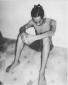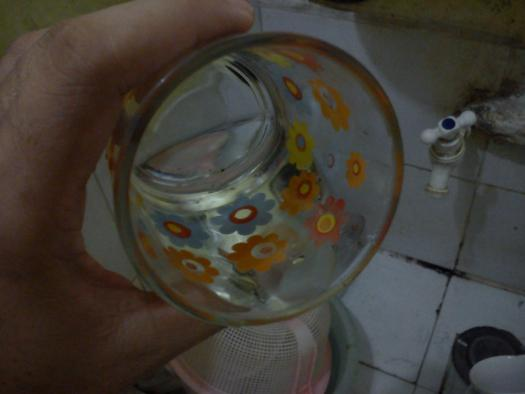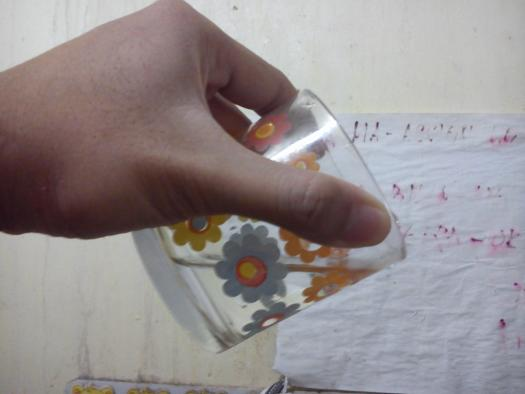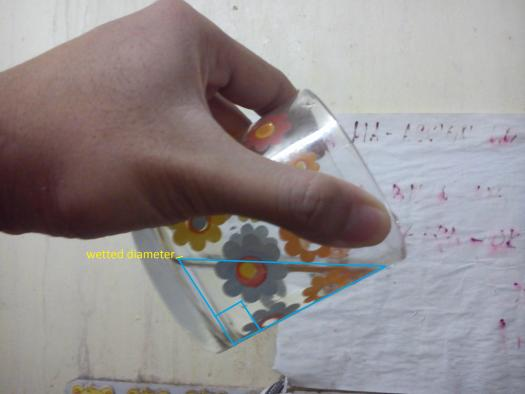# Solid Geometry

2 posts / 0 new
Renz Kolin Gaba...Solid Geometry

From a cylindrical jar 4 in. high and 6 in. in diameter, water is poured by tilting the jar until the center of the bottom is at the surface of the water. A plane is passed through the water parallel to both the axis of the jar and the wetted diameter. What the section made by this plane with the water? If the plane has been passed perpendicular to the wetted diameter, what would be the section?

fitzmerl duron$\mathbf{For \space the \space first \space question:}$

We need a picture to visualize the problem.Looking deeper at the picture, we found out that the section made by a plane running parallel through the cylinder axis and wetted diameter is a:$$\color{blue}{\mathbf{Parabolic \space Segment}}$$

Well...it does look like an ellipse broke into two equal parts though...

$\mathbf{For \space the \space second \space question:}$

We need a picture to visualize the problem.Looking deeper at the picture, we found out that the section made by a plane running perpendicular through the wetted diameter is a:$$\color{blue}{\mathbf{Right \space Triangle}}$$

Alternate solutions are encouraged....

Subscribe to MATHalino on